In [ ]:
import warnings
warnings.filterwarnings('ignore')
warnings.simplefilter('ignore')

In :
%matplotlib notebook
import paramonte as pm
import numpy as np
import scipy as sp
import pandas as pd
import seaborn as sns
import matplotlib as mpl
import matplotlib.pyplot as plt
print('\n'.join(f'{m.__name__} {m.__version__}' for m in globals().values() if getattr(m, '__version__', None)))
sns.set()

paramonte 2.5.1
numpy 1.19.5
scipy 1.5.4
pandas 1.2.0
seaborn 0.11.0
matplotlib 3.3.3


## Sampling a univariate normal distribution via the ParaMonte library's ParaDRAM routine¶

For a more comprehensive discussion of different aspects and attributes of the sampler and how to run the simulation in parallel, see the multivariate Normal distribution Jupyter Notebook example in the same folder as this Jupyter file exists.

Suppose we want to sample random points from a standard univariate Gaussian function. The following Python function getLogFunc() returns the natural logarithm of the Probability Density Function of the univariate standard Gaussian distribution.

In :
import numpy as np
logSqrt2Pi = np.log(np.sqrt(2*np.pi))
def getLogFunc(x): return -0.5*x**2 - logSqrt2Pi


Since the mathematical objective functions (e.g., probability density functions) can take extremely small or large values, we often work with their natural logarithms instead. This is the reason behind the naming convention used in the ParaMonte library for the user's objective functions: getLogFunc, implying that the user must provide a function that returns the natural logarithm of the target objective function.

See this Jupyter Notebook for an in-depth discussion of why we need to work with the logarithm of mathematical objective functions in optimization and sampling problems.

We will sample random points from this objective function by calling the ParaDRAM sampler (Delayed-Rejection Adaptive Metropolis-Hastings Markov Chain Monte Carlo sampler) of the ParaMonte library.

The simplest scenario would be to run the simulation with the default specifications that are appropriately determined by the ParaDRAM sampler.

To run the sampler in parallel, you will have to first save the MPI-enabled script as an external file. Visit the ParaMonte library's documentation website for more information, or see this parallel ParaDRAM simulation Jupyter notebook example.

However, for further clarity of this particular example, we will specify an output folder for the automatically-named output files of the simulation.

In :
import paramonte as pm

print( pm.version.interface.get() )
print( pm.version.kernel.get() )

pm.checkForUpdate() # check for new versions of the ParaMonte library

pmpd = pm.ParaDRAM()         # define a ParaMonte sampler instance
pmpd.spec.chainSize = 10000  # request a much smaller sample size than the default value (100000)
pmpd.spec.randomSeed = 31951 # initialize the random seed to generate reproducible results
pmpd.spec.overwriteRequested = True # overwrite existing old simulation files with the same names
pmpd.spec.outputFileName = "./out/gaussian" # output files prefix

pmpd.runSampler ( ndim = 1
, getLogFunc = getLogFunc
)

ParaMonte Python Interface Version 2.5.1
ParaMonte Python Kernel Version 1.5.1

ParaMonte - NOTE: You have the latest version of the ParaMonte library.
ParaMonte - NOTE: To see the most recent changes to the library, visit,
ParaMonte - NOTE:
ParaMonte - NOTE:     https://www.cdslab.org/paramonte/notes/overview/paramonte-python-release-notes

ParaDRAM - NOTE: To run the ParaDRAM sampler in parallel mode visit:
ParaDRAM - NOTE: If you are using Jupyter notebook, check the Jupyter's
ParaDRAM - NOTE: terminal window for realtime simulation progress and report.

ParaDRAM - NOTE: where you should replace pmpd with your ParaDRAM sampler's object name.



The realtime simulation progress information will printed on your Anaconda prompt window (NOT inside your Jupyter notebook). Once the simulation is finished, the ParaDRAM routine generates 5 output files, each of which contains information about certain aspects of the simulation, all available here to view.

In :
pmpd.readSample()
sample = pmpd.sampleList # shorten the component name to work with.

ParaDRAM - WARNING: The delimiter is neither given as input to readSample()
ParaDRAM - WARNING: nor set as a simulation specification of the ParaDRAM object.
ParaDRAM - WARNING: This information is essential, otherwise how could the output files be parsed?
ParaDRAM - WARNING: For now, the ParaDRAM sampler will assume a comma-separated
ParaDRAM - WARNING: file format for the contents of the sample file(s) to be parsed.

ParaDRAM - NOTE: 1 files detected matching the pattern: "./out/gaussian*_sample.txt"

ParaDRAM - NOTE: ndim = 1, count = 2971
ParaDRAM - NOTE: parsing file contents...
ParaDRAM - NOTE: computing the sample correlation matrix...
ParaDRAM - NOTE: creating a heatmap plot object from scratch... done in 0.018002 seconds.
ParaDRAM - NOTE: computing the sample covariance matrix...
ParaDRAM - NOTE: creating a heatmap plot object from scratch... done in 0.027017 seconds.
ParaDRAM - NOTE: computing the sample autocorrelations...
ParaDRAM - NOTE: creating a line plot object from scratch... done in 0.0 seconds.
ParaDRAM - NOTE: creating a scatter plot object from scratch... done in 0.001001 seconds.
ParaDRAM - NOTE: creating a lineScatter plot object from scratch... done in 0.0 seconds.
ParaDRAM - NOTE: creating a line plot object from scratch... done in 0.0 seconds.
ParaDRAM - NOTE: creating a scatter plot object from scratch... done in 0.0 seconds.
ParaDRAM - NOTE: creating a lineScatter plot object from scratch... done in 0.0 seconds.
ParaDRAM - NOTE: creating a line3 plot object from scratch... done in 0.0 seconds.
ParaDRAM - NOTE: creating a scatter3 plot object from scratch... done in 0.0 seconds.
ParaDRAM - NOTE: creating a lineScatter3 plot object from scratch... done in 0.0 seconds.
ParaDRAM - NOTE: creating a jointplot plot object from scratch... done in 0.0 seconds.
ParaDRAM - NOTE: creating a histplot plot object from scratch... done in 0.0 seconds.
ParaDRAM - NOTE: creating a kdeplot1 plot object from scratch... done in 0.0 seconds.
ParaDRAM - NOTE: creating a kdeplot2 plot object from scratch... done in 0.0 seconds.
ParaDRAM - NOTE: creating a contour3 plot object from scratch... done in 0.0 seconds.
ParaDRAM - NOTE: creating a contourf plot object from scratch... done in 0.0 seconds.
ParaDRAM - NOTE: creating a contour plot object from scratch... done in 0.0 seconds.
ParaDRAM - NOTE: creating a grid plot object from scratch... done in 0.001 seconds.

ParaDRAM - NOTE: The processed sample files are now stored in the newly-created
ParaDRAM - NOTE: component sampleList of the ParaDRAM object as a Python list.
ParaDRAM - NOTE: For example, to access the contents of the first (or the only) sample file, try:
ParaDRAM - NOTE: where you will have to replace pmpd with your ParaDRAM instance name.
ParaDRAM - NOTE: To access the plotting tools, try:
ParaDRAM - NOTE:     pmpd.sampleList.plot.<PRESS TAB TO SEE THE LIST OF PLOTS>
ParaDRAM - NOTE:     pmpd.sampleList.plot.line()         # to make 2D line plots.
ParaDRAM - NOTE:     pmpd.sampleList.plot.scatter()      # to make 2D scatter plots.
ParaDRAM - NOTE:     pmpd.sampleList.plot.lineScatter()  # to make 2D line-scatter plots.
ParaDRAM - NOTE:     pmpd.sampleList.plot.line3()        # to make 3D line plots.
ParaDRAM - NOTE:     pmpd.sampleList.plot.scatter3()     # to make 3D scatter plots.
ParaDRAM - NOTE:     pmpd.sampleList.plot.lineScatter3() # to make 3D line-scatter plots.
ParaDRAM - NOTE:     pmpd.sampleList.plot.contour()      # to make fast 2D kernel density plots.
ParaDRAM - NOTE:     pmpd.sampleList.plot.contourf()     # to make fast 2D kernel density filled contour plots.
ParaDRAM - NOTE:     pmpd.sampleList.plot.contour3()     # to make fast 3D kernel density contour plots.
ParaDRAM - NOTE:     pmpd.sampleList.plot.histplot()     # to make seaborn 1D distribution plots.
ParaDRAM - NOTE:     pmpd.sampleList.plot.kdeplot1()     # to make seaborn 1D kernel density plots.
ParaDRAM - NOTE:     pmpd.sampleList.plot.kdeplot2()     # to make seaborn 2D kernel density plots.
ParaDRAM - NOTE:     pmpd.sampleList.plot.grid()         # to make GridPlot
ParaDRAM - NOTE: To plot or inspect the variable autocorrelations or the correlation/covariance matrices, try:
ParaDRAM - NOTE:     pmpd.sampleList.stats.<PRESS TAB TO SEE THE LIST OF COMPONENTS>



To quickly visualize the generated sample as a histogram, try,

In :
sample.plot.histplot()

ParaDRAM - NOTE: making the histplot plot...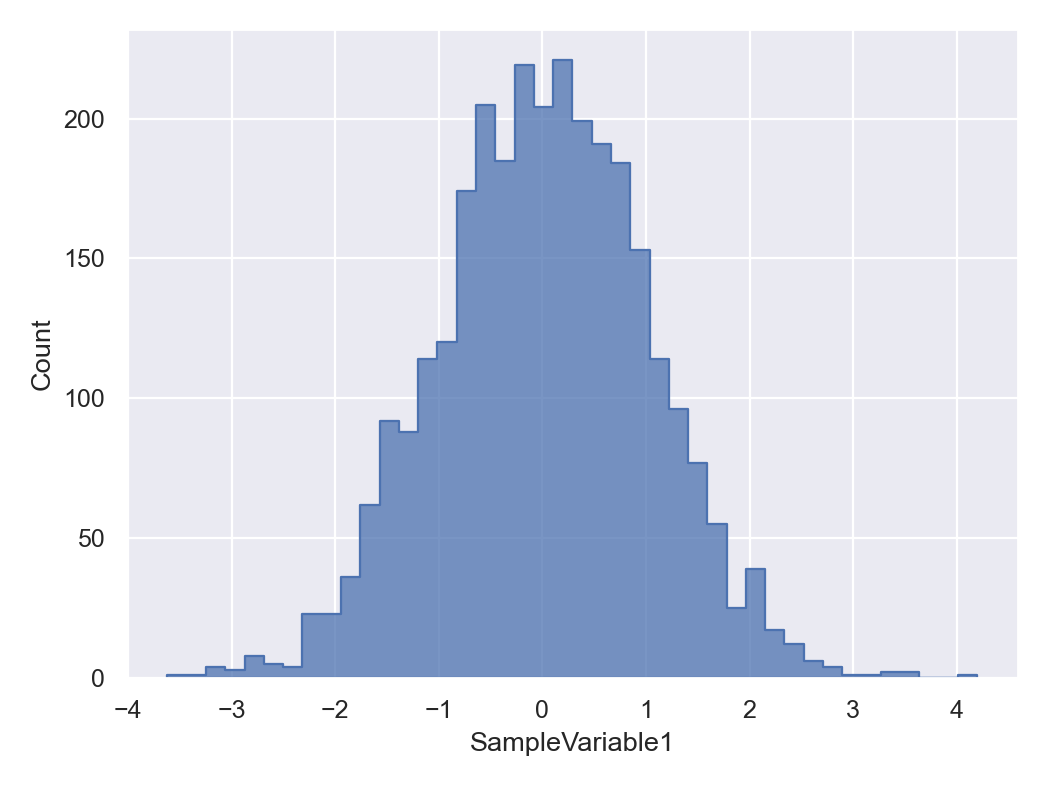done in 0.146003 seconds.


If the variable names are specified for the sampler before running the simulations, the sampler will automatically assign names to each variable. To change the x-label, for example, you can try the following,

In :
sample.plot.histplot()
sample.plot.histplot.currentFig.axes.set_xlabel("Standard Gaussian Random Value")

ParaDRAM - NOTE: making the histplot plot...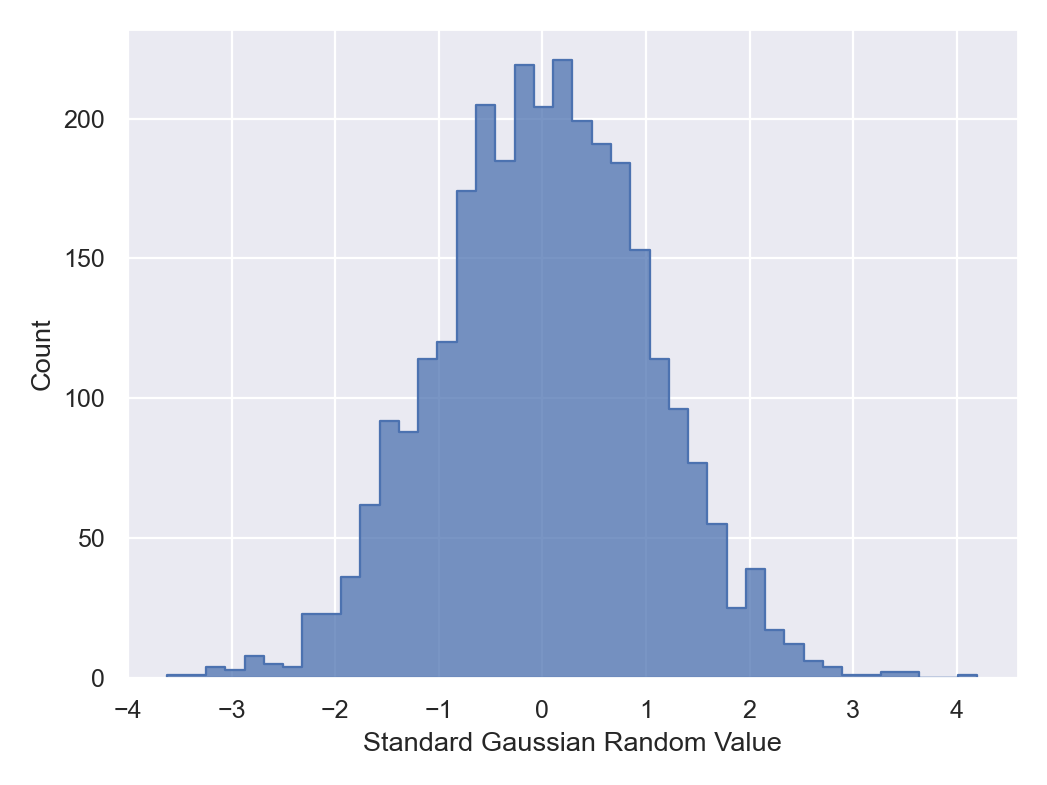done in 0.121961 seconds.

Out:
Text(0.5, 31.07499999999998, 'Standard Gaussian Random Value')

We can also add target values to the plot (we can also reset the plot to the default settings, which we do here),

In :
sample.plot.histplot.reset()
sample.plot.histplot()
sample.plot.histplot.currentFig.axes.set_xlabel("Standard Gaussian Random Value")
sample.plot.histplot.target() # add a line corresponding to the maxLogFunc (mode) of the sampled points.

ParaDRAM - NOTE: making the histplot plot...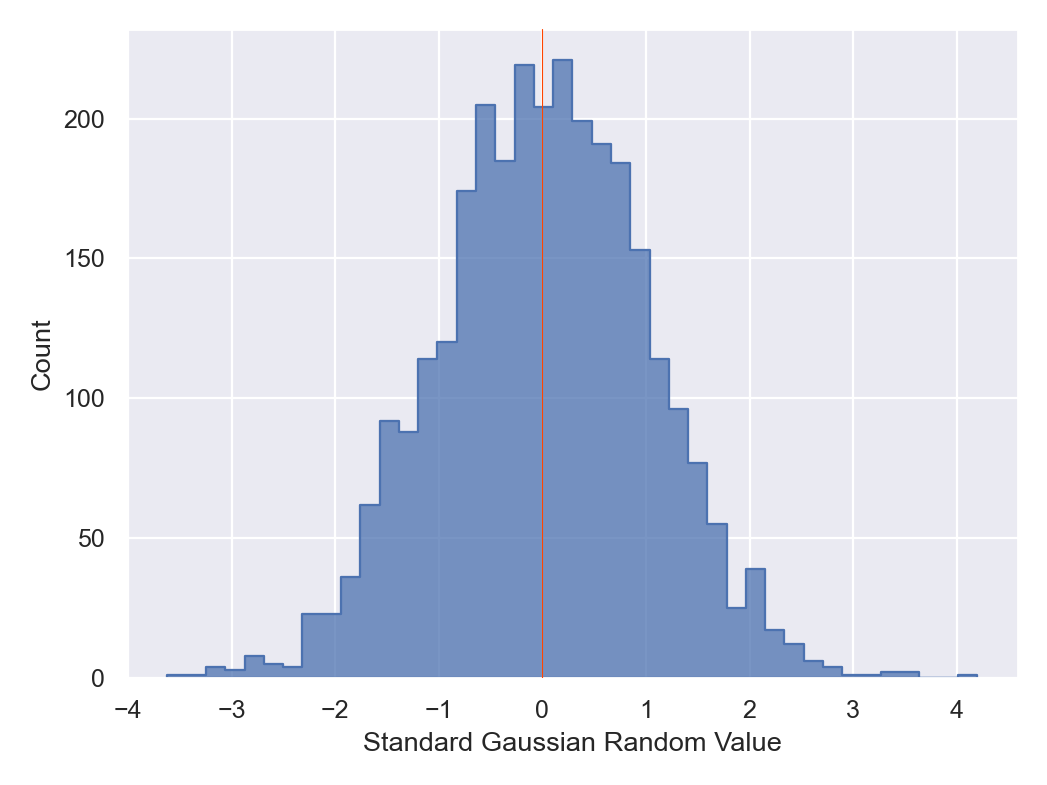done in 0.113999 seconds.


By default, if no target value is specified, the mode of the sampled states will be used the target value. We can also add any other value of interest. For example, let's add the 1-sigma standard deviation lines to the plot,

In :
sample.plot.histplot.reset()
sample.plot.histplot()
sample.plot.histplot.currentFig.axes.set_xlabel("Standard Gaussian Random Value")

avg = sample.df["SampleVariable1"].mean()
sample.plot.histplot.target( value = avg )

std = sample.df["SampleVariable1"].std()
sample.plot.histplot.target.axvline.kws.linestyle = "--"
sample.plot.histplot.target( value = avg - std )
sample.plot.histplot.target( value = avg + std )

ParaDRAM - NOTE: making the histplot plot...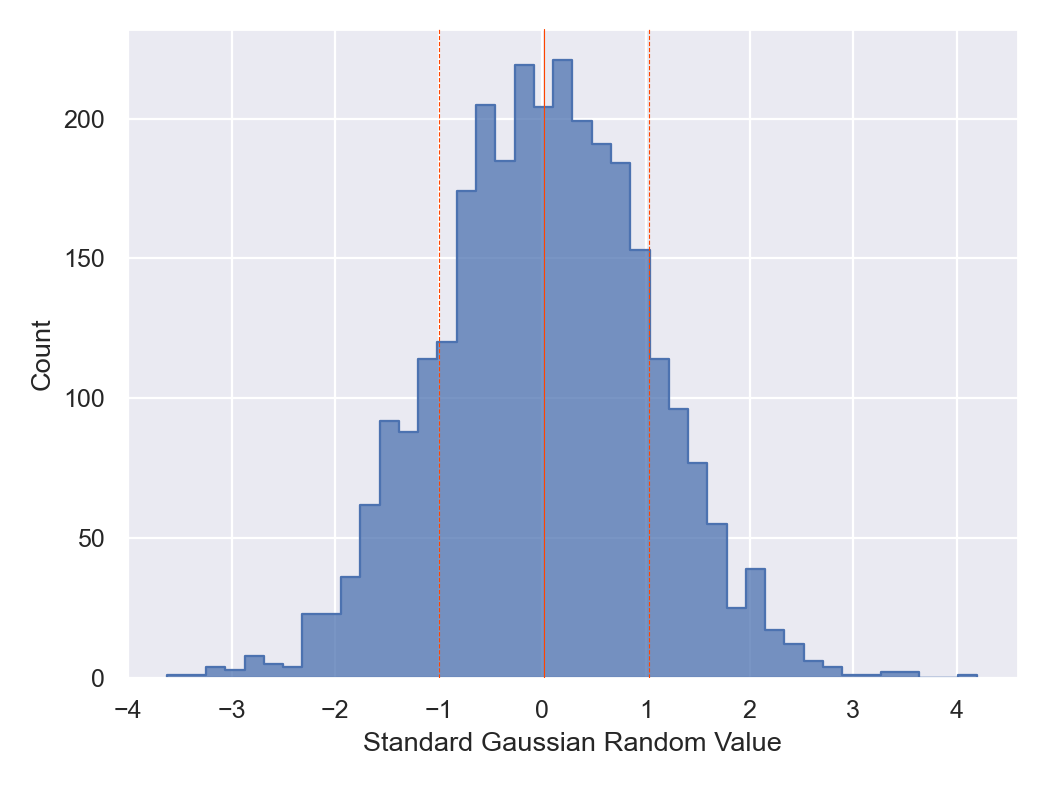done in 0.105999 seconds.


In the above figure, we are now showing the mean and the 1-sigma distribution of the sampled points around the mean.

To make a trace-plot of the sample, try,

In :
sample.plot.line()

ParaDRAM - NOTE: making the line plot...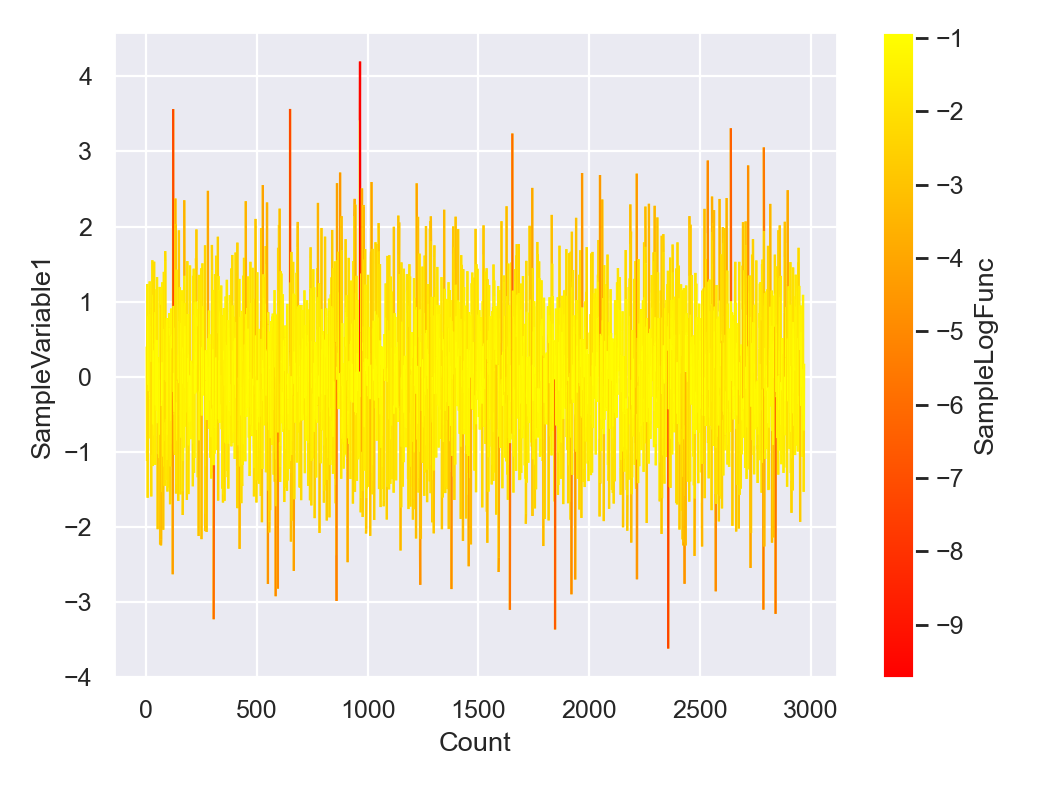done in 0.183967 seconds.


To change the scale of the x-axis, try,

In :
sample.plot.line()
sample.plot.line.currentFig.axes.set_xscale("log")

ParaDRAM - NOTE: making the line plot...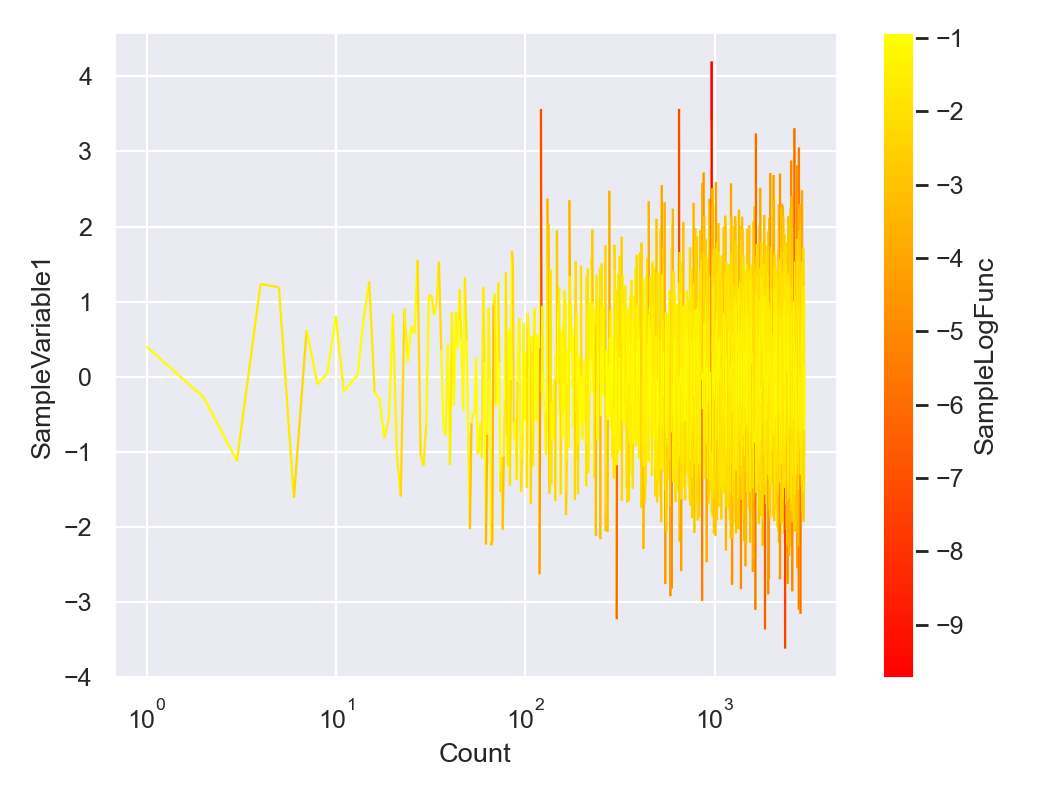done in 0.168003 seconds.


By default, the color of the line in the trace-plot will represent the value returned by getLogFunc() at the given sampled point. To turn the color off, you can instead try,

In :
sample.plot.line.ccolumns

Out:
'SampleLogFunc'
In :
sample.plot.line.ccolumns = None
sample.plot.line()

ParaDRAM - NOTE: making the line plot...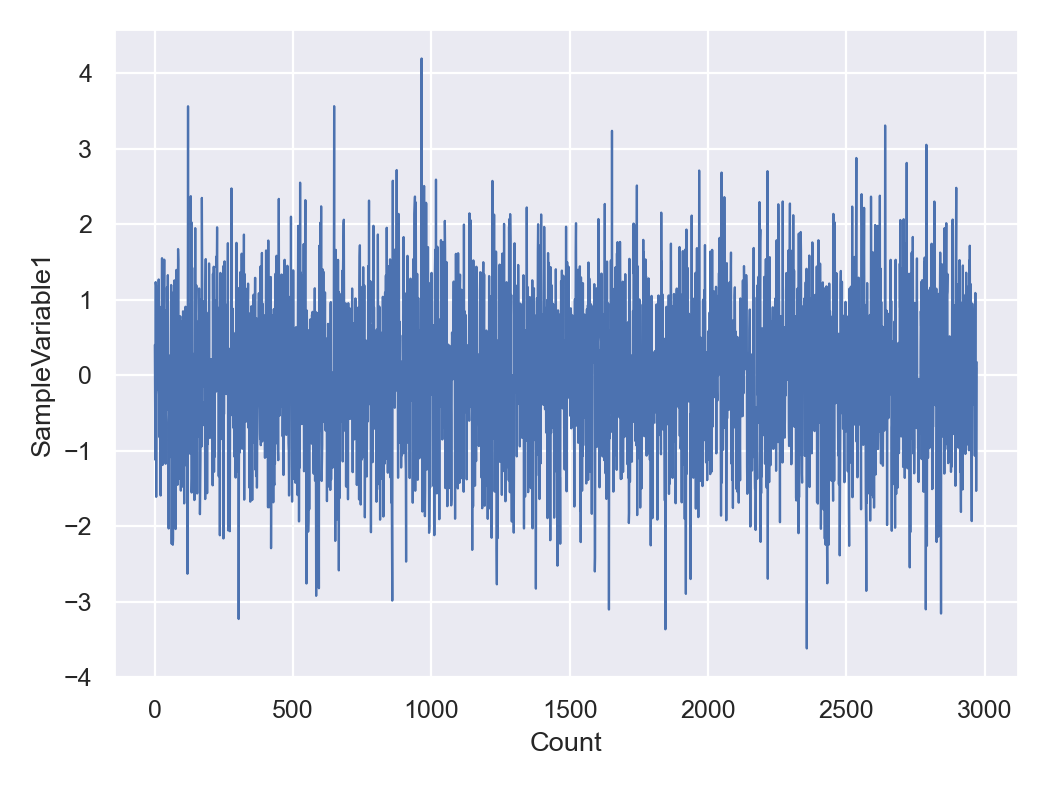done in 0.097 seconds.


There are many other properties of the plot that can be set or modified via the attributes of the pmpd.sampleList.plot.line object. To see them all, see the documentation of the object,

In :
sample.plot.line.helpme()

Here is the help information on the LineScatterPlot class:

This is the LineScatterPlot class for generating instances
of line or scatter plots or the combination of the two in
two or three dimensions based on the visualization tools
of the matplotlib and seaborn Python libraries.

**Usage**

First generate an object of this class by optionally
passing the following parameters described below. Then call
the make() method. The generated object is also callable
with the same input parameters as the object's constructor.

**Parameters**

plotType

A string indicating the name of the plot to be constructed.

dataFrame (optional)

A pandas dataFrame whose data will be plotted.

methodName (optional)

The name of the ParaMonte sample requesting the BasePlot.

reportEnabled (optional)

A boolean whose value indicates whether guidelines should be
printed in the standard output.

resetPlot (optional)

A function that resets the properties of the plot as desired
from outside. If provided, a pointer to this function will be
saved for future internal usage.

**Attributes**

xcolumns

An attribute that determines the columns of dataFrame
to be visualized as the X-axis. It can have three forms:

1.  A list of column indices in dataFrame.
2.  A list of column names in dataFrame.columns.
3.  A range(start,stop,step) of column indices.

Examples:

1.  xcolumns = [0,1,4,3]
2.  xcolumns = ["SampleLogFunc","SampleVariable1"]
3.  xcolumns = range(17,7,-2)

The default behavior includes all columns of the dataFrame.

ycolumns

An attribute that determines the columns of dataFrame
to be visualized as the Y-axis. It can have three forms:

1.  A list of column indices in dataFrame.
2.  A list of column names in dataFrame.columns.
3.  A range(start,stop,step) of column indices.

Examples:

1.  ycolumns = [0,1,4,3]
2.  ycolumns = ["SampleLogFunc","SampleVariable1"]
3.  ycolumns = range(17,7,-2)

The default behavior includes all columns of the dataFrame.

zcolumns (exists only in 3D plot objects)

An attribute that determines the columns of dataFrame
to be visualized as the Z-axis. It can have three forms:

1.  A list of column indices in dataFrame.
2.  A list of column names in dataFrame.columns.
3.  A range(start,stop,step) of column indices.

Examples:

1.  zcolumns = [0,1,4,3]
2.  zcolumns = ["SampleLogFunc","SampleVariable1"]
3.  zcolumns = range(17,7,-2)

The default behavior includes all columns of the dataFrame.

ccolumns

An attribute that determines the columns of dataFrame
to be used for color mapping. It can have three forms:

1.  A list of column indices in dataFrame.
2.  A list of column names in dataFrame.columns.
3.  A range(start,stop,step) of column indices.

Examples:

1.  ccolumns = [0,1,4,3]
2.  ccolumns = ["SampleLogFunc","SampleVariable1"]
3.  ccolumns = range(17,7,-2)

If ccolumns is set to None, then no color-mapping
will be made. If it is set to an empty list [], then
the values from the rows attribute will be used for
color-mapping.

rows

An attribute that determines the rows of dataFrame to be
visualized. It can be either:

1.  A range(start,stop,step), or,
2.  A list of row indices in dataFrame.index.

Examples:

1.  rows = range(17,7,-2)
2.  rows = [i for i in range(7,17)]

The default behavior includes all rows of the dataFrame.

plot (exists only for line or lineScatter plots in 2D and 3D)

A structure with two attributes:

enabled

A boolean indicating whether a call to the plot()
function of the matplotlib library should be made
or not.

kws

A structure whose components are directly passed as
keyword arguments to the plot() function.

Example usage:

.. code-block:: python

plot.enabled = True
plot.kws.linewidth = 1

**NOTE**

If a desired property is missing among the kws
attributes, simply add the field and its value to
the component.

scatter (exists only for scatter / lineScatter plots in 2D and 3D)

A structure with two attributes:

enabled

A boolean indicating whether a call to the
scatter() function of the matplotlib library

kws

A structure whose components are directly passed as
keyword arguments to the scatter() function.

Example usage:

.. code-block:: python

scatter.enabled = True
scatter.kws.s = 2

**NOTE**

If a desired property is missing among the kws
attributes, simply add the field and its value to
the component.

lineCollection (exists only for 2D / 3D line / lineScatter plots)

A structure with two attributes:

enabled

A boolean indicating whether a call to the
LineCollection() class of the matplotlib
library should be made or not. This will result
in line plots that are color-mapped.

kws

A structure whose components are directly passed as
keyword arguments to the LineCollection() class
of matplotlib library.

Example usage:

.. code-block:: python

lineCollection.enabled = True
lineCollection.kws.linewidth = 1

**NOTE**

If a desired property is missing among the kws
attributes, simply add the field and its value to
the component.

set

A structure with two attributes:

enabled

A boolean indicating whether a call to the set()
function of the seaborn library should be made or not.

kws

A structure whose components are directly passed as
keyword arguments to the set() function.

Example usage:

.. code-block:: python

set.kws.style = "darkgrid"

**NOTE**

If a desired property is missing among the kws
attributes, simply add the field and its value to
the component.

axes (available only in 1D and 2D plots)

A structure with one attribute:

kws

A structure whose components are directly passed as
keyword arguments to the gca() function of the
matplotlib library.

Example usage:

.. code-block:: python

axes.kws.facecolor = "w"

**NOTE**

If a desired property is missing among the kws
attributes, simply add the field and its value to
the component.

axes3d (available only in 3D plots)

A structure with one attribute:

kws

A structure whose components are directly passed as
keyword arguments to the Axes3D() function of the
matplotlib library.

Example usage:

.. code-block:: python

axes3d.kws.facecolor = "w"

**NOTE**

If a desired property is missing among the kws
attributes, simply add the field and its value to
the component.

figure

A structure with two attributes:

enabled

A boolean indicating whether a call to the figure()
function of the matplotlib library should be made or not.
If a call is made, a new figure will be generated.
Otherwise, the current active figure will be used.

kws

A structure whose components are directly passed as
keyword arguments to the figure() function.

Example usage:

.. code-block:: python

figure.kws.facecolor = "w"

**NOTE**

If a desired property is missing among the kws
attributes, simply add the field and its value to
the component.

colorbar (exists only for plots that require colorbar)

A structure with two attributes:

enabled

A boolean indicating whether a call to the colorbar()
function of the matplotlib library should be made or not.
If a call is made, a new figure will be generated.
Otherwise, the current active figure will be used.

kws

A structure whose components are directly passed as
keyword arguments to the colorbar() function of
the matplotlib library.

**NOTE**

If a desired property is missing among the kws
attributes, simply add the field and its value to
the component.

A colorbar will be added to a plot only if a color-mappings
is requested in the plot.

legend (may not exist for some types of plots)

A structure with two attributes:

enabled

A boolean indicating whether a call to the legend()
function of the matplotlib library should be made or not.
If a call is made, a new figure will be generated.
Otherwise, the current